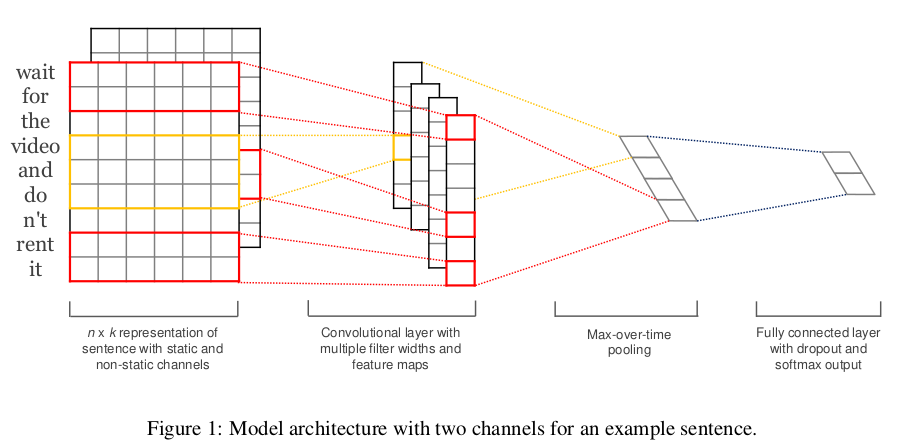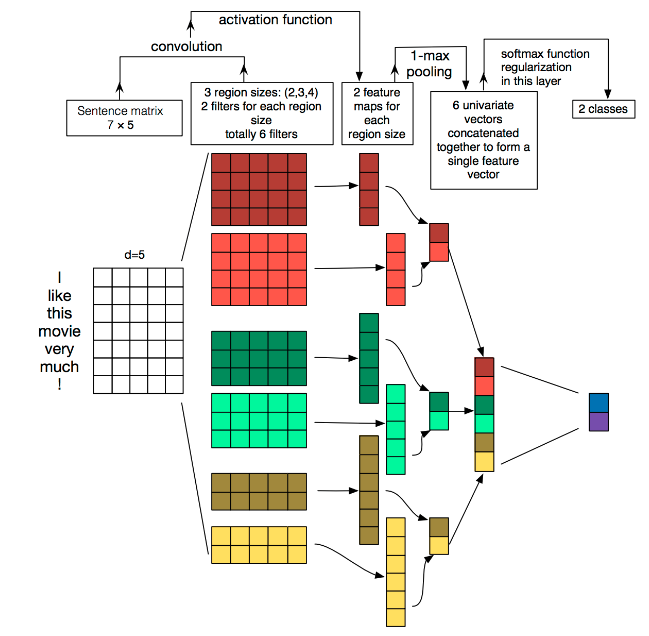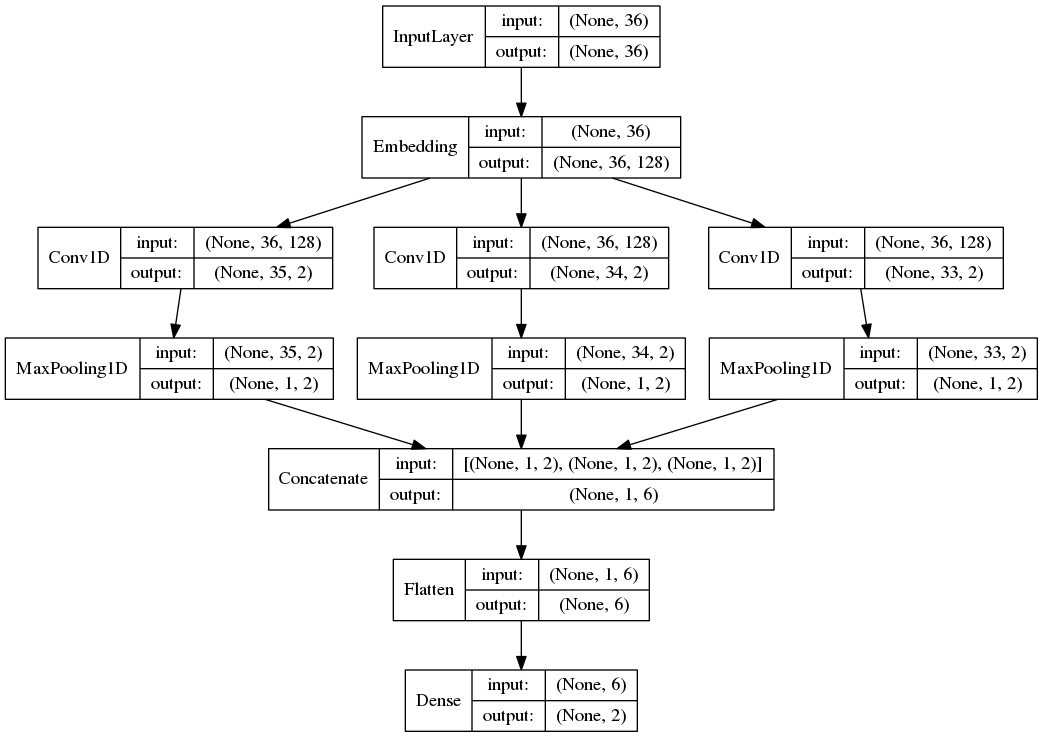# [NLP] TextCNN模型原理和实现

## 1. 模型原理

### 1.1 论文

Yoon Kim在论文(2014 EMNLP) Convolutional Neural Networks for Sentence Classification提出TextCNN。

### 1.2 网络结构TextCNN的详细过程原理图如下：TextCNN详细过程：

• Embedding：第一层是图中最左边的7乘5的句子矩阵，每行是词向量，维度=5，这个可以类比为图像中的原始像素点。
• Convolution：然后经过 kernel_sizes=(2,3,4) 的一维卷积层，每个kernel_size 有两个输出 channel。
• MaxPolling：第三层是一个1-max pooling层，这样不同长度句子经过pooling层之后都能变成定长的表示。
• FullConnection and Softmax：最后接一层全连接的 softmax 层，输出每个类别的概率。

• 图像中可以利用 (R, G, B) 作为不同channel；
• 文本的输入的channel通常是不同方式的embedding方式（比如 word2vec或Glove），实践中也有利用静态词向量和fine-tunning词向量作为不同channel的做法。

• 图像是二维数据；
• 文本是一维数据，因此在TextCNN卷积用的是一维卷积（在word-level上是一维卷积；虽然文本经过词向量表达后是二维数据，但是在embedding-level上的二维卷积没有意义）。一维卷积带来的问题是需要通过设计不同 kernel_size 的 filter 获取不同宽度的视野

Pooling层：

“我觉得这个地方景色还不错，但是人也实在太多了”

虽然前半部分体现情感是正向的，全局文本表达的是偏负面的情感，利用 k-max pooling能够很好捕捉这类信息。

## 2. 实现

import logging

from keras import Input
from keras.layers import Conv1D, MaxPool1D, Dense, Flatten, concatenate, Embedding
from keras.models import Model
from keras.utils import plot_model

def textcnn(max_sequence_length, max_token_num, embedding_dim, output_dim, model_img_path=None, embedding_matrix=None):
""" TextCNN: 1. embedding layers, 2.convolution layer, 3.max-pooling, 4.softmax layer. """
x_input = Input(shape=(max_sequence_length,))
logging.info("x_input.shape: %s" % str(x_input.shape))  # (?, 60)

if embedding_matrix is None:
x_emb = Embedding(input_dim=max_token_num, output_dim=embedding_dim, input_length=max_sequence_length)(x_input)
else:
x_emb = Embedding(input_dim=max_token_num, output_dim=embedding_dim, input_length=max_sequence_length,
weights=[embedding_matrix], trainable=True)(x_input)
logging.info("x_emb.shape: %s" % str(x_emb.shape))  # (?, 60, 300)

pool_output = []
kernel_sizes = [2, 3, 4]
for kernel_size in kernel_sizes:
c = Conv1D(filters=2, kernel_size=kernel_size, strides=1)(x_emb)
p = MaxPool1D(pool_size=int(c.shape))(c)
pool_output.append(p)
logging.info("kernel_size: %s \t c.shape: %s \t p.shape: %s" % (kernel_size, str(c.shape), str(p.shape)))
pool_output = concatenate([p for p in pool_output])
logging.info("pool_output.shape: %s" % str(pool_output.shape))  # (?, 1, 6)

x_flatten = Flatten()(pool_output)  # (?, 6)
y = Dense(output_dim, activation='softmax')(x_flatten)  # (?, 2)
logging.info("y.shape: %s \n" % str(y.shape))

model = Model([x_input], outputs=[y])
if model_img_path:
plot_model(model, to_file=model_img_path, show_shapes=True, show_layer_names=False)
model.summary()
return model

• 数据量较大：可以直接随机初始化embeddings，然后基于语料通过训练模型网络来对embeddings进行更新和学习。
• 数据量较小：可以利用外部语料来预训练(pre-train)词向量，然后输入到Embedding层，用预训练的词向量矩阵初始化embeddings。（通过设置weights=[embedding_matrix]）。
• 静态(static)方式训练过程中不再更新embeddings。实质上属于迁移学习，特别是在目标领域数据量比较小的情况下，采用静态的词向量效果也不错。（通过设置trainable=False）
• 非静态(non-static)方式在训练过程中对embeddings进行更新和微调(fine tune)，能加速收敛。（通过设置trainable=True）

plot_model()画出的TextCNN模型结构图如下：posted @ 2018-09-20 14:52  焦距  阅读(...)  评论(...编辑  收藏